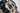# How to Type Deduction

• Compilers

Type deduction seems daunting but the solution is simple enough: constraint negotiation, this has the side effect of performing variable resolution and all for you too.

## #Problem

A couple of days ago I was hacking away, making progress on VSL when I realized I had a big problem: Type Deduction. Now basic type deduction as which is featured in C/C++, Java, and pretty much all other typed languages is not a problem but there were cases like:

func add(a: Int, b: Double) -> Double {
return Double(a) + b;
}

func add(a: Double, b: Double) -> Int {
return Int(a) + Int(b)
}

let a = add(a: add(a: 1, b: 2), b: 3)

Looking at this you might observe some difficulties in the fact that:

• Integers can represent multiple types (Int8, UInt, Double, Float, etc.)
• The outer add must have have matching types for add(1, 2) and 3
• The types a could be are ambiguous (or are they?)

So this here is a classic constraint-problem and I’ll show how VSL solves this problem. The exact implementation may depend on your type system, is it lazy? Are bindings strongly typed?

## #Solution?

The solution is simple enough. Constraint negotiation, this has the side effect of performing variable resolution and all for you but again, it’s simple enough.

The core of this is your intersection. This may seem obvious but the negotiation nature is key to why this works.

### #The algorithm

I’ll try to simplify as much as possible but let’s have a really basic example for our purposes:

let num: Int = 1

Looking at this we know somethings:

• They are two ‘typable’ things, 1 and num.
• num has a constraint (it’s type must be Int)

So we can take 1 and num and look at what their types are at the beginning:

1. 1: can be Int, UInt, Int8, etc.
2. num: must be Int

okay, done! right? –no.

First we need to distinguish the kinds of types we can have:

• Applied Types
• Candidate Types

In this scenario Int (of num) is our applied type, and a Int, UInt, Int8, ... (of 1) are our candidate types. In this scenario we only have one applied type but we can easily have more. Examples are when we have:

func f(a: Int) -> Double { ... }
func f(a: Double) -> Float { ... }

print( f(a: f(a: 1)) )

In this case they are applied candidates for f(a: 1). (Double, Float) but we are able to deduce that calls the f(a: Int) -> Double because if it were the second, the other function call would not have a valid type signature.

Let’s describe this:

\begin{aligned} \alpha &= \text{set of applied types} \\ \gamma &= \text{set of candidate types} \end{aligned}

Normally you’d think we’d do $N_\alpha \cap N_\gamma$ but this works on the idea that:

$\Delta\gamma\vdash N_{\gamma}=P_{T}(N)_\gamma\Leftrightarrow|P_{T}(N)_{\alpha}|>0$

When you mutate $C_A$, the parent should be the same if it has type candidates. This allows propagation of constraints and deferred deduction of these. This means we can do:

let a      = 1 // Ambiguous type
let b: Int = a // a must be Int

if $\text{a node}_\gamma = \text{b node}_\gamma$ then when we apply the constraint^[Terminology: this type of constraint is called a ‘context-constraint’. This is compared to constraints which we deduce, meaning the user has explicitly specified this constraint.] for b: Int. That would apply to both a and b.

### #Negotiation

Let’s represent a negotiation function with xi $\xi_n$, and the type of constraint we’d negotiate. Each typable node can be represented as a tuple of the graph node $N$ and the negotiations it has $\Xi$:

$t(N)=\left(N,\Xi\right)$

Now every node isn’t going to have a negotiation for every node. So we could define $\Xi$ as:

$t(N)_{\Xi} = t(P_T(N))_{\Xi}$